ISEE Upper Level Quantitative : Circles

Example Questions

Example Question #6 : How To Find Circumference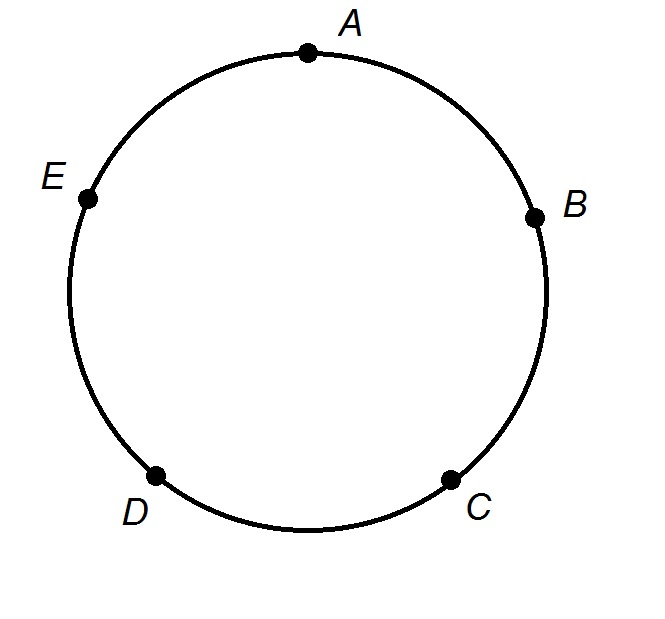The track at Monroe High School is a perfect circle of radius 600 feet, and is shown in the above figure.

Jerry begins his one-mile run at Point A, then runs counterclockwise around the track. At the end of his one-mile run, which is the greater quantity?

(a) The additional distance he would have to run if he were to continue to run counterclockwise to Point A.

(b) The additional distance he would have to run if he were to turn back and run clockwise to Point A.

(b) is greater

It is impossible to tell from the information given

(a) and (b) are equal

(a) is greater

(a) is greater

Explanation:

The circumference of a circle with radius 600 feet is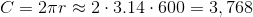feet.

A one mile run would be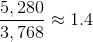times the length of the track.

Therefore, Jerry's run takes him around the track once, and about 0.4 times the length of the track. Since he is running counterclockwise, but has not made it halfway around the track yet, the longer of the two paths is to proceed counterclockwise and run the remaining 0.6 of the track. This makes (a) greater.

Example Question #7 : How To Find CircumferenceThe track at Simon Bolivar High School is a perfect circle of radius 500 feet, and is shown in the above figure. Manuel starts at point C, runs around the track counterclockwise three times, and continues to run clockwise until he makes it to point D. Which of the following comes closest to the number of miles Manuel has run?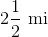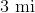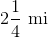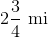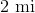Explanation:

The circumference of a circle with radius 500 feet is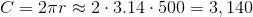feet.

Manuel runs this distance three times, then he runs from Point C to D, which is about four-fifths of this distance. Therefore, Manuel's run will be about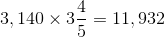feet.

Divide by 5,280 to convert to miles: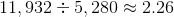,

making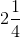miles the response closest to the actual running distance.

Example Question #8 : How To Find Circumference

The radii of six circles form an arithmetic sequence. The radius of the second-smallest circle is twice that of the smallest circle. Which of the following, if either, is the greater quantity?

(a) The circumference of the largest circle

(b) Twice the circumference of the third-smallest circle

(b) is greater

(a) and (b) are equal

It is impossible to tell from the information given

(a) is greater

(a) and (b) are equal

Explanation:

Call the radius of the smallest circle. The radius of the second-smallest circle is then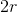, and the common difference of the radii is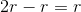.

The radii of the six circles are, from least to greatest: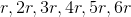The largest circle has circumference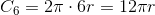The third-smallest circle has circumference: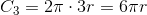Twice this is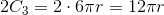The circumference of the sixth circle is equal to twice that of the third-smallest  circle, so the correct choice is that that (a) and (b) are equal.

Example Question #9 : How To Find Circumference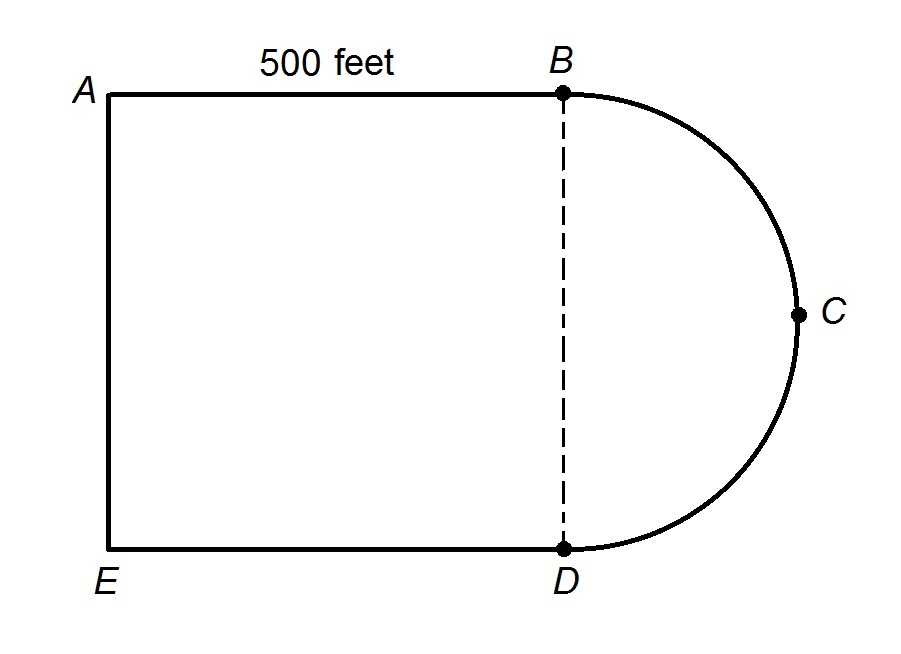The track at Truman High School is shown above; it is comprised of a square and a semicircle.

Veronica begins at Point A, runs three times around the track counterclockwise, and continues until she reaches Point B. Which of the following comes closest to the distance Veronica runs?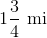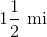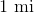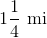Explanation:

First, it is necessary to know the length of the semicircle connecting Points B and D, which has diameter 500 feet; this length is about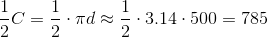feet.

The distance around the track is about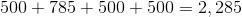feet.

Veronica runs around the track three complete times, for a distance of about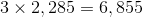feet.

She then runs from Point A to Point E, which is another 500 feet; Point E to Point D, which is yet another 500 feet, and, finally Point D to Point B, for a final 785 feet. The total distance Veronica runs is about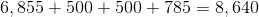feet.

Divide by 5,280 to convert to miles: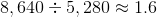The closest answer is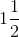miles.

Example Question #10 : How To Find CircumferenceThe track at Monroe High School is a perfect circle of radius 600 feet, and is shown in the above figure. Quinnella wants to run around the track for one and a half miles. If Quinnella starts at point C and runs counterclockwise, which of the following is closest to the point at which she will stop running?

(Assume the five points are evenly spaced)

Between Points B and C

Between Points C and D

Between Points A and B

Between Points D and E

Between Points E and A

Between Points B and C

Explanation:

A circle of radius 600 feet will have a circumference offeet.

Quinnella will run one and a half miles, or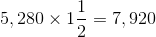feet,

which is about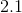times the circumference of the circle.

Quinnella will run around the track twice, returning to Point C; she will not quite make it to Point B a third time, since that is one-fifth of the track, or 0.2. The correct response is that she will be between Points B and C.

Example Question #161 : Plane Geometry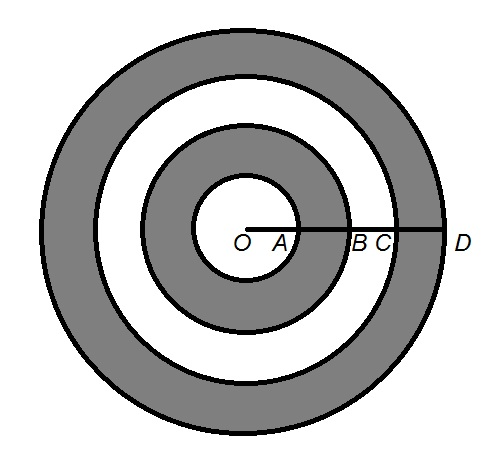In the above figure,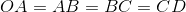.

Which is the greater quantity?

(a) The sum of the circumferences of the inner and outer circles

(b) The sum of the circumferences of the second-largest and third-largest circles

It is impossible to determine which is greater from the information given

(a) is the greater quantity

(a) and (b) are equal

(b) is the greater quantity

(a) and (b) are equal

Explanation:

For the sake of simplicity, we will assume that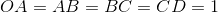; this reasoning is independent of the actual length.

The four concentric circles have radii 1, 2, 3, and 4, respectively, and their circumferences can be found by multiplying these radii by.

The inner and outer circles have circumferences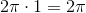and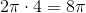, respectively; the sum of these circumferences is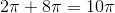. The other two circles have circumferences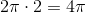and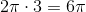; the sum of these circumferences is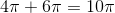.

The two sums are therefore equal.

Example Question #1 : Sectors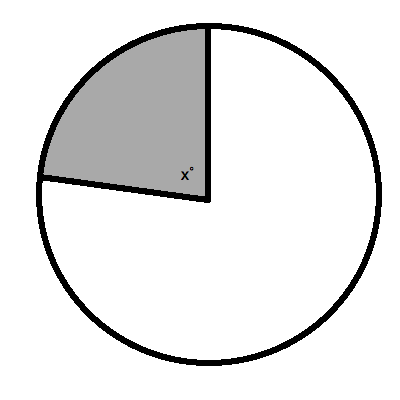What is the angle measure ofin the figure above if the sector comprises 37% of the circle?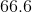˚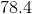˚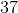˚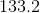˚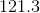˚˚

Explanation:

It is very easy to compute the angle of a sector if we know what it is as a percentage of the total circle.  To do this, you merely need to multiply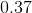by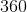˚.  This yields˚.

Example Question #2 : SectorsWhat is the angle measure ofin the figure above if the sector comprises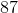% of the circle?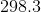˚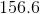˚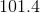˚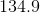˚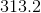˚˚

Explanation:

It is very easy to compute the angle of a sector if we know what it is as a percentage of the total circle.  To do this, you merely need to multiply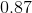by˚.  This yields˚.

Example Question #3 : Sectors

What is the angle measure ofin the figure if the sector comprises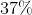of the circle?˚˚˚˚˚˚

Explanation:

It is very easy to compute the angle of a sector if we know what it is as a percentage of the total circle.  To do this, you merely need to multiplyby˚.  This yields˚

Example Question #4 : Sectors

Circle A has twice the radius of Circle B. Which is the greater quantity?

(a) The area of asector of Circle A

(b) The area of Circle B

(a) is greater.

(b) is greater.

It is impossible to tell from the information given.

(a) and (b) are equal.

Letbe the radius of Circle B. The radius of Circle A is therefore.sector of a circle comprises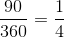of the circle. Thesector of circle A has area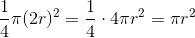, the area of Circle B. The two quantities are equal.